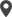Enable Dark Mode!By: Hridhya D

# How to Add a Search Filter for Computed Fields in Odoo 15

## Technical Odoo 15

First of all, we need to know why we use computed fields in Odoo. Computed fields are used when we want to get computed/calculated values from some other fields we use computed fields. i.e., values for a field can be calculated using functions instead of reading a value stored in a database. An example of the computed field is when we want to calculate the total amount of some product and that amount we get by multiplying the total number of products and the product price. I.e., Total = number of products x price of the product.
In Odoo, by Default Compute Fields are not stored in the database.
So, there may be situations where we need to search or filter based on these computed fields.
In order to do that, in this blog, we will be discussing mainly how to Add a Search Function for a Non-Stored Compute Field in Odoo. There are two ways to store the computed fields in Odoo. We can make the field store by adding store=True. And another method is by using a search function set it on the field.
Let me explain this by giving an example. So, if we want to filter based on the visa that has expired. For that, we have added an expiration_date field which is a Date field, Is_expired field which is a Boolean field. As you can see in the code given below.
`expiration_date = fields.Date(string = "Expiration Date")is_expired = fields.Boolean(string = 'Is Expired', compute = '_compute_is_expired', search = '_search_is_expired')`
Here, in the is_expired field, we have added a search function along with the computing field. In the python code given below, we have defined the compute and the search function. In the search function, we have to pass 3 arguments i.e., self, operator, and value.
`def _compute_is_expired(self):    now = fields.Datetime.now()for rec in self:    rec.is_expired = now.date() > rec.expiration_datedef _search_is_expired(self, operator, value):    now = fields.Datetime.now()ids = self.env['visa.approval'].search([('expiration_date', '<', now)])return [('id', 'in', ids)]`
In the XML, we have the filter as you can see in the below code, and also defined the search_view_id. Record id of the search view is given as the reference of the search_view_id.
`<record model="ir.ui.view" id="view_expired_search">    <field name="name">visa.approval.search</field>    <field name="model">visa.approval</field>    <field name="arch" type="xml">        <search string="Visa">            <filter name="is_expired" string="Is Expired" domain="[('is_expired', '=', True)]" />        </search>    </field></record><record id="visa_approval_action" model="ir.actions.act_window">    <field name="name">Visa Approval</field>    <field name="type">ir.actions.act_window</field>    <field name="res_model">visa.approval</field>    <field name="view_mode">tree,form</field>    <field name="search_view_id" ref="view_expired_search" /></record>`
Similarly, we can add a search function for computed fields in different types of fields, not only for boolean fields.
The second method to search based on a computed field is to add store=True. In the same example given above, we have added store=True instead of defining the search function. And also wrote the compute function.
`is_expired = fields.Boolean(string='Is Expired', compute='_compute_is_expired', store='True')def _compute_is_expired(self):    now = fields.Datetime.now()for rec in self:    rec.is_expired = now.date() > rec.expiration_date`
This is how we can add a search filter for computed fields in odoo.In the compute function, we will be writing code of how we compute the value. And search function defines which is to be returned. When it comes to store=True, it saves the data to the database.

If you need any assistance in odoo, we are online, please chat with us.

### Recent Posts

#### How to Use Jmeter for Performance TestingCalicut

Cybrosys Technologies Pvt. Ltd.
Neospace, Kinfra Techno Park
Kakkancherry, Calicut
Kerala, India - 673635London

Cybrosys Limited
Alpha House,
100 Borough High Street, London,
SE1 1LB, United KingdomKochi

Cybrosys Technologies Pvt. Ltd.
1st Floor, Thapasya Building,
Kochi, India - 682030.Bangalore

Cybrosys Techno Solutions
The Estate, 8th Floor,